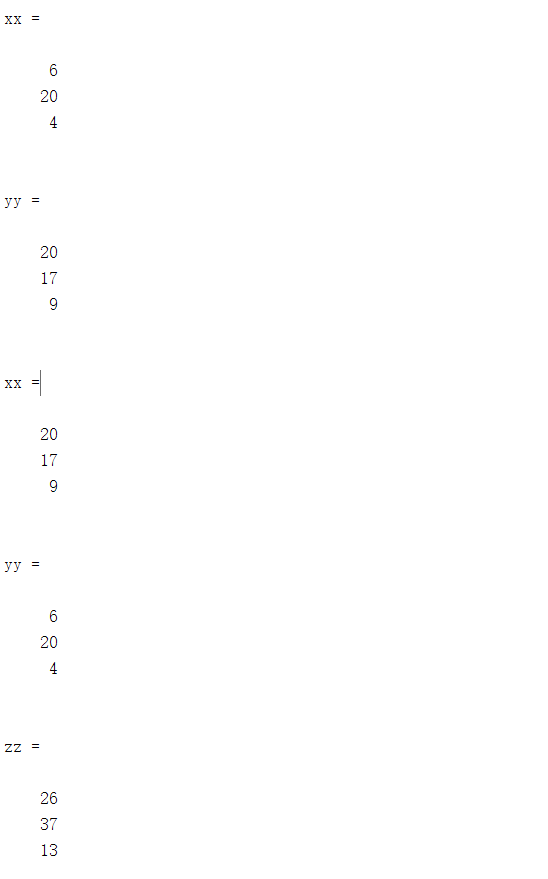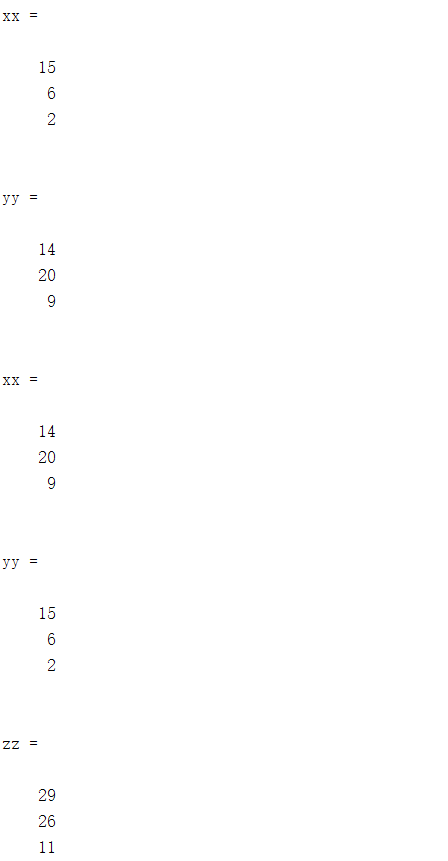9 篇文章 0 订阅

# 二、下面是一个简单的例子

现在需要将xx矩阵和yy矩阵的元素全部交换，并且二者相加得到一个新矩阵zz

## 1.主函数：

execute_2.m

clc
clear
%定义xx，yy,zz矩阵大小
m=3;
n=1;
xx=zeros(m,n);
yy=zeros(m,n);
zz=zeros(m,n);
%初始化xx,yy
xx=init_x(xx,m,n)
yy=init_y(yy,m,n)
%交换xx矩阵和yy矩阵的元素，并求出xx和yy相加的新矩阵zz
[xx,yy,zz]=function_2(xx,yy,zz,m,n)


## 2.初始化xx和yy的函数

init_x.m

function x=init_x(x,m,n)

for i=1:m
for j=1:n
x(i,j)=randsample(20,1);
end
end

init_y.m

function y=init_y(y,m,n)

for i=1:m
for j=1:n
y(i,j)=randsample(20,1);
end
end

## 3.交换矩阵元素以及相加函数

function_2.m

function [x,y,z]=function_2(x,y,z,m,n)
tempx=x;%中间变量
%x和y交换
for i=1:m
for j=1:n
x(i,j)=y(i,j);
y(i,j)=tempx(i,j);
end
end

%x加上y
for i=1:m
for j=1:n
z(i,j)=x(i,j)+y(i,j);
end
end

# 三、  结果截图03-1684104-13361
03-16431
03-161060
04-02867
10-013195
02-133万+
11-031307
03-2187
10-072万+
12-221333
04-192931
10-12231
12-1235万+点击重新获取扫码支付余额充值